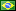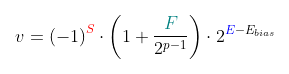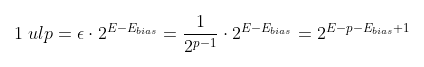###Author Topic: An important quantity for floating point calculations.  (Read 529 times)

####fredericopissarra

• Full Member
•• Posts: 352
• Country:##### An important quantity for floating point calculations.
« on: June 26, 2023, 08:00:20 PM »
Since "floating point" is a fraction between 2 unsigned integer values multiplied by a scale factor, if that scale factor have an expoent zero, the minimum step between one value and another is 1/2^(p-1) -- where p is the precision, in bits. This comes from the formula and structure of floating point, accordingly to IEEE-754:This value is known as EPSILON (the 'e' greek letter - this forum don't allow me to post unicode chars!). EPSILON is this minimum step within the scale 2^0 (or between 1 and 2, excluding 2). But we have to consider the scale to calculate the minimum step, given the scale. This minimum scale, multiplying EPSILON by the scale is known as "unit at last position" (ulp):An example: Consider a float with p=24 and a value: 10^7. This value has scale of 2^26:
Code: [Select]
`e=floor(log2(10^7))=26`Or, the value is between 2^26 (67108864) and 2^27 (134217728) -- E - Ebias=26.
The minimum step is:
Code: [Select]
`1 ulp = 2^(26-24+1) = 2^3 = 8.`The value 100000000 is representable since is divisible by 8 (1 ulp), [10^7/8 -> q=1250000, remainder=0], but the previous value is, necessarily 9999992 and the next, 10000008. No values in between can be exactly represented. If you try to do:
Code: [Select]
`float f = 10e7f;f = f + 1.0f;`The result is, still, 10e7! Is you try to add 5 (> 1/2 ulp), the final value will be rounded to 10000008. This is ok because operations use an extra "guard" bit to improve rounding.

The bigger the value, bigger the scale factor and bigger the "error" (1 ulp).

Even worse: Because of the ulp the algebraic commutative property is lost. a + b + c isn't always equal to a + c + b. An example:
Code: [Select]
`float a, b, c;a = 10e7f;b = -10e7f;c = 1;r = a + b + c;  // 10^7 - 10^7 + 1 = (10^7 - 10^7) + 1 = 0 + 1 = 1s = a + c + b;  // 10^7 + 1 - 10^7 = (10^7 + 1) - 10^7 = 10^7 - 10^7 = 0`Try yourself...
« Last Edit: June 26, 2023, 08:10:34 PM by fredericopissarra »

####Deskman243

• Jr. Member
•• Posts: 46##### Re: An important quantity for floating point calculations.
« Reply #1 on: June 27, 2023, 04:11:38 PM »
These are pretty fun post because they remind me of all the times we take for granted the CPU computational aspects. For me I learned naturally from many resources online why programmers strive for quintessentials like conjecture builds for Linear Algebra or Calculus. Common sense reminds us that long numbers are big and is why advocates for electronics spend alot of money on new microprocessors. As a programmer while I confess I don't have the enthusiasm for calculus on daily assembly programs (convolutions are not easy!) I do believe one day we can all achieve beyond the electronic barrier of patents (sorry I check posts from IEEE too haha).

The closest form answers I imagine here are similarly congruent discrete transforms.There are a handful of denominational branches here and I would really quickly suggest algorithmic research texts or courses as a reference being that these are very common relations in most Computer related fields and conventions. If there was anything specific you would like to respond to I would be really happy to continue your conversation.
« Last Edit: June 27, 2023, 04:19:34 PM by Deskman243 »